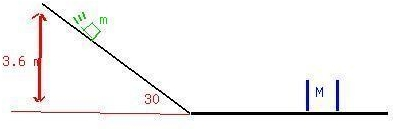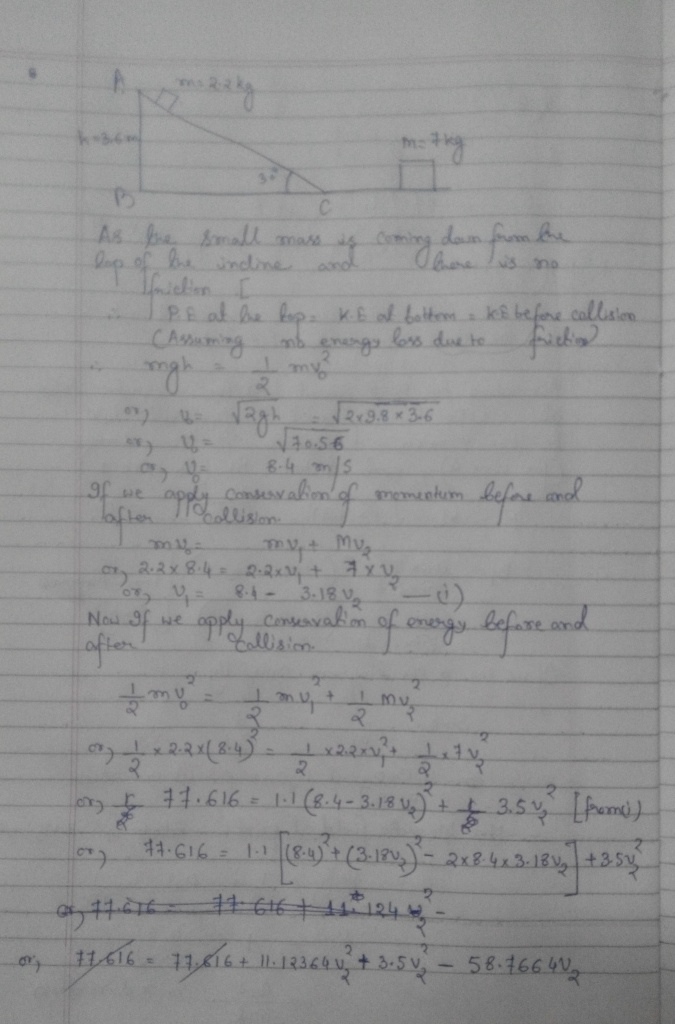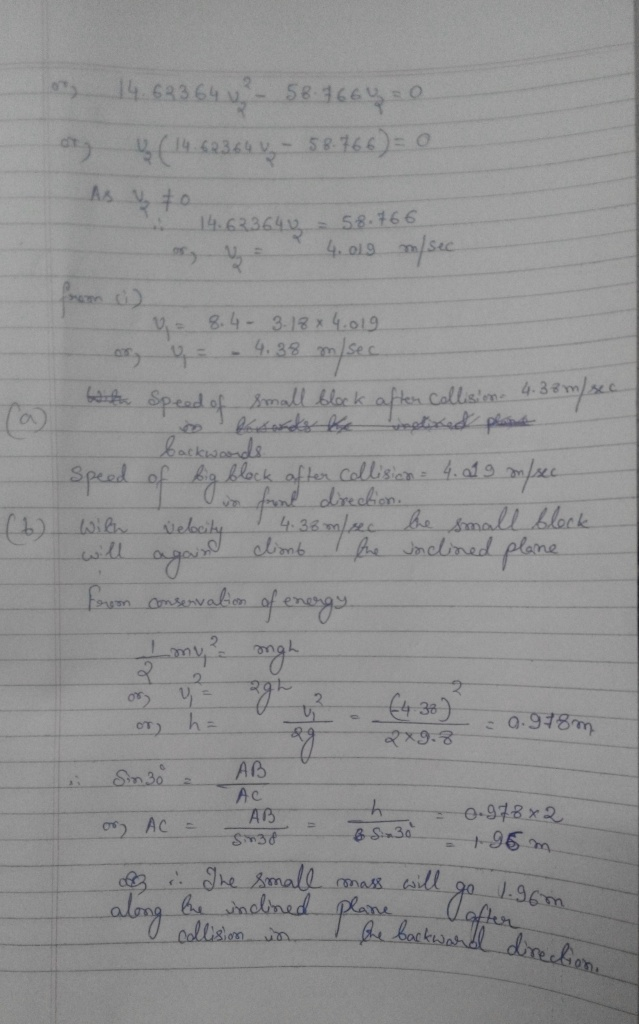# A block of mass m=2.20 kg slides down a 30 degree incline which is 3.60 m high.emancipezN 2021-03-07 Answered

A block of mass m=2.20 kg slides down a 30 degree incline which is 3.60 m high. At the bottom, it strikes a block of mass=7.00 kg which is at rest on a horizontal surface in the picture. If the collision is elastic, and friction can be ignored, determine (a)the speeds of the two blocks after the collision, and (b) how far back up the incline the smaller mass will go.You can still ask an expert for help

• Questions are typically answered in as fast as 30 minutes

Solve your problem for the price of one coffee

• Math expert for every subject
• Pay only if we can solve itottcomn

Step 1Step 2